xCombinatory logicEncyclopedia
Combinatory logic is a notation introduced by Moses Schönfinkel
Moses Schönfinkel
Moses Ilyich Schönfinkel, also known as Moisei Isai'evich Sheinfinkel , was a Russian logician and mathematician, known for the invention of combinatory logic.- Life :Schönfinkel attended the Novorossiysk University of Odessa, studying mathematics under Samuil Osipovich...

Haskell Brooks Curry was an American mathematician and logician. Curry is best known for his work in combinatory logic; while the initial concept of combinatory logic was based on a single paper by Moses Schönfinkel, much of the development was done by Curry. Curry is also known for Curry's...

to eliminate the need for variables
Variable (mathematics)
In mathematics, a variable is a value that may change within the scope of a given problem or set of operations. In contrast, a constant is a value that remains unchanged, though often unknown or undetermined. The concepts of constants and variables are fundamental to many areas of mathematics and...

in mathematical logic
Mathematical logic
Mathematical logic is a subfield of mathematics with close connections to foundations of mathematics, theoretical computer science and philosophical logic. The field includes both the mathematical study of logic and the applications of formal logic to other areas of mathematics...

. It has more recently been used in computer science
Computer science
Computer science or computing science is the study of the theoretical foundations of information and computation and of practical techniques for their implementation and application in computer systems...

as a theoretical model of computation
Computation
Computation is defined as any type of calculation. Also defined as use of computer technology in Information processing.Computation is a process following a well-defined model understood and expressed in an algorithm, protocol, network topology, etc...

and also as a basis for the design of functional programming languages. It is based on combinators. A combinator is a higher-order function
Higher-order function
In mathematics and computer science, higher-order functions, functional forms, or functionals are functions which do at least one of the following:*take one or more functions as an input*output a function...

that uses only function application and earlier defined combinators to define a result from its arguments.

## Combinatory logic in mathematics

Combinatory logic was originally intended as a 'pre-logic' that would clarify the role of quantified variables in logic, essentially by eliminating them. Another way of eliminating quantified variables is Quine's
Willard Van Orman Quine
Willard Van Orman Quine was an American philosopher and logician in the analytic tradition...

predicate functor logic
Predicate functor logic
In mathematical logic, predicate functor logic is one of several ways to express first-order logic by purely algebraic means, i.e., without quantified variables. PFL employs a small number of algebraic devices called predicate functors that operate on terms to yield terms...

. While the expressive power
Expressive power
In computer science, the expressive power of a language describes the ideas expressible in that language.For example, the Web Ontology Language expression language profile lacks ideas which can be expressed in OWL2 RL . OWL2 EL may therefore be said to have less expressive power than OWL2 RL...

of combinatory logic typically exceeds that of first-order logic
First-order logic
First-order logic is a formal logical system used in mathematics, philosophy, linguistics, and computer science. It goes by many names, including: first-order predicate calculus, the lower predicate calculus, quantification theory, and predicate logic...

, the expressive power of predicate functor logic
Predicate functor logic
In mathematical logic, predicate functor logic is one of several ways to express first-order logic by purely algebraic means, i.e., without quantified variables. PFL employs a small number of algebraic devices called predicate functors that operate on terms to yield terms...

is identical to that of first order logic (Quine 1960, 1966, 1976).

The original inventor of combinatory logic, Moses Schönfinkel
Moses Schönfinkel
Moses Ilyich Schönfinkel, also known as Moisei Isai'evich Sheinfinkel , was a Russian logician and mathematician, known for the invention of combinatory logic.- Life :Schönfinkel attended the Novorossiysk University of Odessa, studying mathematics under Samuil Osipovich...

, published nothing on combinatory logic after his original 1924 paper, and largely ceased to publish after Joseph Stalin
Joseph Stalin
Joseph Vissarionovich Stalin was the Premier of the Soviet Union from 6 May 1941 to 5 March 1953. He was among the Bolshevik revolutionaries who brought about the October Revolution and had held the position of first General Secretary of the Communist Party of the Soviet Union's Central Committee...

consolidated his power in 1929. Curry rediscovered the combinators while working as an instructor at the Princeton University
Princeton University
Princeton University is a private research university located in Princeton, New Jersey, United States. The school is one of the eight universities of the Ivy League, and is one of the nine Colonial Colleges founded before the American Revolution....

in late 1927. In the latter 1930s, Alonzo Church
Alonzo Church
Alonzo Church was an American mathematician and logician who made major contributions to mathematical logic and the foundations of theoretical computer science. He is best known for the lambda calculus, Church–Turing thesis, Frege–Church ontology, and the Church–Rosser theorem.-Life:Alonzo Church...

and his students at Princeton
Princeton University
Princeton University is a private research university located in Princeton, New Jersey, United States. The school is one of the eight universities of the Ivy League, and is one of the nine Colonial Colleges founded before the American Revolution....

invented a rival formalism for functional abstraction, the lambda calculus
Lambda calculus
In mathematical logic and computer science, lambda calculus, also written as λ-calculus, is a formal system for function definition, function application and recursion. The portion of lambda calculus relevant to computation is now called the untyped lambda calculus...

, which proved more popular than combinatory logic. The upshot of these historical contingencies was that until theoretical computer science began taking an interest in combinatory logic in the 1960s and 70s, nearly all work on the subject was by Haskell Curry
Haskell Brooks Curry was an American mathematician and logician. Curry is best known for his work in combinatory logic; while the initial concept of combinatory logic was based on a single paper by Moses Schönfinkel, much of the development was done by Curry. Curry is also known for Curry's...

and his students, or by Robert Feys
Robert Feys
Robert Feys was a Belgian logician and philosopher, who worked at the University of Leuven .In 1958 Feys and Haskell B. Curry devised the type inference algorithm for the simply typed lambda calculus ....

in Belgium
Belgium
Belgium , officially the Kingdom of Belgium, is a federal state in Western Europe. It is a founding member of the European Union and hosts the EU's headquarters, and those of several other major international organisations such as NATO.Belgium is also a member of, or affiliated to, many...

. Curry and Feys (1958), and Curry et al. (1972) survey the early history of combinatory logic. For a more modern parallel treatment of combinatory logic and the lambda calculus, see Barendregt
Henk Barendregt
Hendrik Pieter Barendregt is a Dutch logician, known for his work in lambda calculus and type theory.Barendregt studied mathematical logic at Utrecht University, obtaining his Masters in 1968 and his Ph.D. in 1971, both cum laude, under Dirk van Dalen and Georg Kreisel...

(1984), who also reviews the models
Model theory
In mathematics, model theory is the study of mathematical structures using tools from mathematical logic....

Dana Scott
Dana Scott
Dana Stewart Scott is the emeritus Hillman University Professor of Computer Science, Philosophy, and Mathematical Logic at Carnegie Mellon University; he is now retired and lives in Berkeley, California...

devised for combinatory logic in the 1960s and 70s.

## Combinatory logic in computing

In computer science
Computer science
Computer science or computing science is the study of the theoretical foundations of information and computation and of practical techniques for their implementation and application in computer systems...

, combinatory logic is used as a simplified model of computation
Computation
Computation is defined as any type of calculation. Also defined as use of computer technology in Information processing.Computation is a process following a well-defined model understood and expressed in an algorithm, protocol, network topology, etc...

, used in computability theory
Computability theory
Computability theory, also called recursion theory, is a branch of mathematical logic that originated in the 1930s with the study of computable functions and Turing degrees. The field has grown to include the study of generalized computability and definability...

and proof theory
Proof theory
Proof theory is a branch of mathematical logic that represents proofs as formal mathematical objects, facilitating their analysis by mathematical techniques. Proofs are typically presented as inductively-defined data structures such as plain lists, boxed lists, or trees, which are constructed...

. Despite its simplicity, combinatory logic captures many essential features of computation.

Combinatory logic can be viewed as a variant of the lambda calculus
Lambda calculus
In mathematical logic and computer science, lambda calculus, also written as λ-calculus, is a formal system for function definition, function application and recursion. The portion of lambda calculus relevant to computation is now called the untyped lambda calculus...

, in which lambda expressions (representing functional abstraction) are replaced by a limited set of combinators, primitive functions from which bound variables are absent. It is easy to transform lambda expressions into combinator expressions, and combinator reduction is much simpler than lambda reduction. Hence combinatory logic has been used to model some non-strict functional programming
Functional programming
In computer science, functional programming is a programming paradigm that treats computation as the evaluation of mathematical functions and avoids state and mutable data. It emphasizes the application of functions, in contrast to the imperative programming style, which emphasizes changes in state...

languages and hardware
Graph reduction machine
A graph reduction machine is a special-purpose computer built to perform combinator calculations by graph reduction.Examples include the SKIM computer, built at the University of Cambridge Computer Laboratory, and the multiprocessor GRIP computer, built at University College London.- References...

. The purest form of this view is the programming language Unlambda
Unlambda
Unlambda is a minimal, "nearly pure" functional programming language invented by David Madore. It is based on combinatory logic, a version of the lambda calculus that omits the lambda operator. It relies mainly on two built-in functions and an "apply" operator...

, whose sole primitives are the S and K combinators augmented with character input/output. Although not a practical programming language, Unlambda is of some theoretical interest.

Combinatory logic can be given a variety of interpretations. Many early papers by Curry showed how to translate axiom sets for conventional logic into combinatory logic equations (Hindley and Meredith 1990). Dana Scott in the 1960s and 70s showed how to marry model theory
Model theory
In mathematics, model theory is the study of mathematical structures using tools from mathematical logic....

and combinatory logic.

## Summary of the lambda calculus

The lambda calculus is
concerned with objects called lambda-terms, which are strings of
symbols of one of the following forms:
• v
• λv.E1
• (E1 E2)

where v is a variable name drawn from a predefined infinite set of
variable names, and E1 and E2 are lambda-terms.

Terms of the form λv.E1 are called abstractions. The variable v is
called the formal parameter of the abstraction, and E1 is the body
of the abstraction. The term λv.E1 represents the function which, applied
to an argument, binds the formal parameter v to the argument and then
computes the resulting value of E1---that is, it returns E1, with
every occurrence of v replaced by the argument.

Terms of the form (E1 E2) are called applications. Applications model
function invocation or execution: the function represented by E1 is to be
invoked, with E2 as its argument, and the result is computed. If E1
(sometimes called the applicand) is an abstraction, the term may be
reduced: E2, the argument, may be substituted into the body of E1
in place of the formal parameter of E1, and the result is a new lambda
term which is equivalent to the old one. If a lambda term contains no
subterms of the form (λv.E1 E2) then it cannot be reduced, and is said to
be in normal form.

The expression E[v := a] represents the result of taking the term E and replacing all free occurrences of v with a. Thus we write
=> E[v := a]

By convention, we take (a b c d ... z) as short for (...(((a b) c) d) ... z). (i.e., application is left associative.)

The motivation for this definition of reduction is that it captures
the essential behavior of all mathematical functions. For example,
consider the function that computes the square of a number. We might
write
The square of x is x*x

(Using "*" to indicate multiplication.) x here is the formal parameter of the function. To evaluate the square for a particular
argument, say 3, we insert it into the definition in place of the
formal parameter:
The square of 3 is 3*3

To evaluate the resulting expression 3*3, we would have to resort to
our knowledge of multiplication and the number 3. Since any
computation is simply a composition of the evaluation of suitable
functions on suitable primitive arguments, this simple substitution
principle suffices to capture the essential mechanism of computation.
Moreover, in the lambda calculus, notions such as '3' and '*' can be
represented without any need for externally defined primitive
operators or constants. It is possible to identify terms in the
lambda calculus, which, when suitably interpreted, behave like the
number 3 and like the multiplication operator, q.v. Church encoding
Church encoding
In mathematics, Church encoding is a means of embedding data and operators into the lambda calculus, the most familiar form being the Church numerals, a representation of the natural numbers using lambda notation...

.

The lambda calculus is known to be computationally equivalent in power to
many other plausible models for computation (including Turing machine
Turing machine
A Turing machine is a theoretical device that manipulates symbols on a strip of tape according to a table of rules. Despite its simplicity, a Turing machine can be adapted to simulate the logic of any computer algorithm, and is particularly useful in explaining the functions of a CPU inside a...

s); that is, any calculation that can be accomplished in any
of these other models can be expressed in the lambda calculus, and
vice versa. According to the Church-Turing thesis, both models
can express any possible computation.

It is perhaps surprising that lambda-calculus can represent any
conceivable computation using only the simple notions of function
abstraction and application based on simple textual substitution of
terms for variables. But even more remarkable is that abstraction is
not even required. Combinatory logic is a model of computation
equivalent to the lambda calculus, but without abstraction. The advantage
of this is that evaluating expressions in lambda calculus is quite complicated
because the semantics of substitution must be specified with great care to
avoid variable capture problems. In contrast, evaluating expressions in
combinatory logic is much simpler, because there is no notion of substitution.

## Combinatory calculi

Since abstraction is the only way to manufacture functions in the
lambda calculus, something must replace it in the combinatory
calculus. Instead of abstraction, combinatory calculus provides a
limited set of primitive functions out of which other functions may be
built.

### Combinatory terms

A combinatory term has one of the following forms:
• x
• P
• (E1 E2)

where x is a variable, P is one of the primitive functions, and (E1 E2) is the application of combinatory terms E1 and E2. The primitive functions themselves are combinators, or functions that, when seen as lambda terms, contain no free variables.
To shorten the notations, a general convention is that (E1 E2 E3 ... En), or even E1 E2 E3... En, denotes the term (...((E1 E2) E3)... En). This is the same general convention as for multiple application in lambda calculus.

### Reduction in combinatory logic

In combinatory logic, each primitive combinator comes with a reduction rule of the form
= E

where E is a term mentioning only variables from the set x1 ... xn. It is in this way that primitive combinators behave as functions.

### Examples of combinators

The simplest example of a combinator is I, the identity
combinator, defined by
= x

for all terms x. Another simple combinator is K, which
manufactures constant functions: (K x) is the function which,
for any argument, returns x, so we say
y) = x

for all terms x and y. Or, following the convention for
multiple application,
= x

A third combinator is S, which is a generalized version of
application:
= (x z (y z))

S applies x to y after first substituting z into
each of them. Or put another way, x is applied to y inside the
environment z.

Given S and K, I itself is unnecessary, since it can
be built from the other two:
x)
= (S K K x)
= (K x (K x))
= x

for any term x. Note that although ((S K K)
x) = (I x) for any x, (S K K)
itself is not equal to I. We say the terms are extensionally equal. Extensional equality captures the
mathematical notion of the equality of functions: that two functions
are equal if they always produce the same results for the same
arguments. In contrast, the terms themselves, together with the
reduction of primitive combinators, capture the notion of
intensional equality of functions: that two functions are equal
only if they have identical implementations up to the expansion of primitive
combinators when these ones are applied to enough arguments. There are many ways to
implement an identity function; (S K K) and I
are among these ways. (S K S) is yet another. We
will use the word equivalent to indicate extensional equality,
reserving equal for identical combinatorial terms.

A more interesting combinator is the fixed point combinator
Fixed point combinator
In computer science, a fixed-point combinator is a higher-order function that computes a fixed point of other functions. A fixed point of a function f is a value x such that x = f. For example, 0 and 1 are fixed points of the function f = x2, because 0 = 02 and 1 = 12...

or Y combinator, which can be used to implement recursion
Recursion
Recursion is the process of repeating items in a self-similar way. For instance, when the surfaces of two mirrors are exactly parallel with each other the nested images that occur are a form of infinite recursion. The term has a variety of meanings specific to a variety of disciplines ranging from...

.

### Completeness of the S-K basis

It is a perhaps astonishing fact that S and K can be
composed to produce combinators that are extensionally equal to
any lambda term, and therefore, by Church's thesis, to any
computable function whatsoever. The proof is to present a transformation,
T[ ], which converts an arbitrary lambda term into an equivalent
combinator.

T[ ] may be defined as follows:
1. T[x] => x
2. T[(E₁ E₂)] => (T[E₁] T[E₂])
3. T[λx.E] => (K T[E]) (if x does not occur free in E)
4. T[λx.x] => I
5. T[λx.λy.E] => T[λx.T[λy.E]] (if x occurs free in E)
6. T[λx.(E₁ E₂)] => (S T[λx.E₁] T[λx.E₂])

This process is also known as abstraction elimination.

#### Conversion of a lambda term to an equivalent combinatorial term

For example, we will convert the lambda term λx.λy.(y x) to a
combinator:
T[λx.λy.(y x)]
= T[λx.T[λy.(y x)]] (by 5)
= T[λx.(S T[λy.y] T[λy.x])] (by 6)
= T[λx.(S I T[λy.x])] (by 4)
= T[λx.(S I (K x))] (by 3 and 1)
= (S T[λx.(S I)] T[λx.(K x)]) (by 6)
= (S (K (S I)) T[λx.(K x)]) (by 3)
= (S (K (S I)) (S T[λx.K] T[λx.x])) (by 6)
= (S (K (S I)) (S (K K) T[λx.x])) (by 3)
= (S (K (S I)) (S (K K) I)) (by 4)

If we apply this combinator to any two terms x and y, it
reduces as follows:
(S (K (S I)) (S (K K) I) x y)
= (K (S I) x (S (K K) I x) y)
= (S I (S (K K) I x) y)
= (I y (S (K K) I x y))
= (y (S (K K) I x y))
= (y (K K x (I x) y))
= (y (K (I x) y))
= (y (I x))
= (y x)

The combinatory representation, (S (K (S I)) (S (K K) I)) is much
longer than the representation as a lambda term, λx.λy.(y x). This is typical. In general, the T[ ] construction may expand a lambda
term of length n to a combinatorial term of length
Θ
Big O notation
In mathematics, big O notation is used to describe the limiting behavior of a function when the argument tends towards a particular value or infinity, usually in terms of simpler functions. It is a member of a larger family of notations that is called Landau notation, Bachmann-Landau notation, or...

(3n) .

#### Explanation of the T[ ] transformation

The T[ ] transformation is motivated by a desire to eliminate
abstraction. Two special cases, rules 3 and 4, are trivial: λx.x is
clearly equivalent to I, and λx.E is clearly equivalent to
(K T[E]) if x does not appear free in E.

The first two rules are also simple: Variables convert to themselves,
and applications, which are allowed in combinatory terms, are
converted to combinators simply by converting the applicand and the
argument to combinators.

It's rules 5 and 6 that are of interest. Rule 5 simply says that to
convert a complex abstraction to a combinator, we must first convert
its body to a combinator, and then eliminate the abstraction. Rule 6
actually eliminates the abstraction.

λx.(E₁ E₂) is a function which takes an argument, say a, and
substitutes it into the lambda term (E₁ E₂) in place of x,
yielding (E₁ E₂)[x : = a]. But substituting a into (E₁ E₂) in place
of x is just the same as substituting it into both E₁ and E₂, so

(E₁ E₂)[x := a] = (E₁[x := a] E₂[x := a])

(λx.(E₁ E₂) a) = ((λx.E₁ a) (λx.E₂ a))
= (S λx.E₁ λx.E₂ a)
= ((S λx.E₁ λx.E₂) a)

By extensional equality,

λx.(E₁ E2) = (S λx.E₁ λx.E₂)

Therefore, to find a combinator equivalent to λx.(E₁ E₂), it is
sufficient to find a combinator equivalent to (S λx.E₁ λx.E₂), and

(S T[λx.E₁] T[λx.E₂])

evidently fits the bill. E₁ and E₂ each contain strictly fewer
applications than (E₁ E₂), so the recursion must terminate in a lambda
term with no applications at all—either a variable, or a term of the
form λx.E.

#### η-reduction

The combinators generated by the T[ ] transformation can be made
smaller if we take into account the η-reduction rule:

T[λx.(E x)] = T[E] (if x is not free in E)

λx.(E x) is the function which takes an argument, x, and
applies the function E to it; this is extensionally equal to the
function E itself. It is therefore sufficient to convert E to
combinatorial form.

Taking this simplification into account, the example above becomes:

T[λx.λy.(y x)]
= ...
= (S (K (S I)) T[λx.(K x)])
= (S (K (S I)) K) (by η-reduction)

This combinator is equivalent to the earlier, longer one:

(S (K (S I)) K x y)
= (K (S I) x (K x) y)
= (S I (K x) y)
= (I y (K x y))
= (y (K x y))
= (y x)

Similarly, the original version of the T[ ] transformation
transformed the identity function λf.λx.(f x) into (S (S (K S) (S (K K) I)) (K I)). With the η-reduction rule, λf.λx.(f x) is
transformed into I.

#### One-point basis

There are one-point bases from which every combinator can be composed extensionally equal to any lambda term. The simplest example of such a basis is {X} where:

Xλx.((xS)K)

It is not difficult to verify that:
X (X (X X)) =ηβ K and
X (X (X (X X))) =ηβ S.

Since {K, S} is a basis, it follows that {X} is a basis too. The Iota
Iota and Jot
Iota and its successor Jot are Turing tarpits, esoteric programming languages that are designed to be as small as possible but still Turing-complete. Each uses two symbols and involves two operations, with simple denotational semantics defined in terms of lambda calculus...

programming language uses X as its sole combinator.

Another simple example of a one-point basis is:

X' ≡ λx.(x K S K) with
(X' X') X' =β K and
X' (X' X') =β S

X' does not need η contraction in order to produce K and S.

#### Combinators B, C

In addition to S and K, Schönfinkel
Moses Schönfinkel
Moses Ilyich Schönfinkel, also known as Moisei Isai'evich Sheinfinkel , was a Russian logician and mathematician, known for the invention of combinatory logic.- Life :Schönfinkel attended the Novorossiysk University of Odessa, studying mathematics under Samuil Osipovich...

's paper included two combinators which are now called B and C, with the following reductions:

(C f x y) = (f y x)
(B f g x) = (f (g x))

He also explains how they in turn can be expressed using only S and K.

These combinators are extremely useful when translating predicate logic or lambda calculus into combinator expressions. They were also used by Curry
Haskell Brooks Curry was an American mathematician and logician. Curry is best known for his work in combinatory logic; while the initial concept of combinatory logic was based on a single paper by Moses Schönfinkel, much of the development was done by Curry. Curry is also known for Curry's...

, and much later by David Turner
David Turner (computer scientist)
Professor David Turner is a British computer scientist.He has a D.Phil. from the University of Oxford. He has held professorships at Queen Mary College, London, University of Texas at Austin and the University of Kent at Canterbury, where he now retains the post of Emeritus Professor.He is...

, whose name has been associated with their computational use. Using them, we can extend the rules for the transformation as follows:
1. T[x] => x
2. T[(E₁ E₂)] => (T[E₁] T[E₂])
3. T[λx.E] => (K T[E]) (if x is not free in E)
4. T[λx.x] => I
5. T[λx.λy.E] => T[λx.T[λy.E]] (if x is free in E)
6. T[λx.(E₁ E₂)] => (S T[λx.E₁] T[λx.E₂]) (if x is free in both E₁ and E₂)
7. T[λx.(E₁ E₂)] => (C T[λx.E₁] T[E₂]) (if x is free in E₁ but not E₂)
8. T[λx.(E₁ E₂)] => (B T[E₁] T[λx.E₂]) (if x is free in E₂ but not E₁)

Using B and C combinators, the transformation of
λx.λy.(y x) looks like this:

T[λx.λy.(y x)]
= T[λx.T[λy.(y x)]]
= T[λx.(C T[λy.y] x)] (by rule 7)
= T[λx.(C I x)]
= (C I) (η-reduction)
= C*(traditional canonical notation : X* = X I)
= I'(traditional canonical notation: X' = C X)

And indeed, (C I x y) does reduce to (y x):

(C I x y)
= (I y x)
= (y x)

The motivation here is that B and C are limited versions of S.
Whereas S takes a value and substitutes it into both the applicand and
its argument before performing the application, C performs the
substitution only in the applicand, and B only in the argument.

The modern names for the combinators come from Haskell Curry
Haskell Brooks Curry was an American mathematician and logician. Curry is best known for his work in combinatory logic; while the initial concept of combinatory logic was based on a single paper by Moses Schönfinkel, much of the development was done by Curry. Curry is also known for Curry's...

's doctoral thesis of 1930 (see B,C,K,W System
B,C,K,W system
The B, C, K, W system is a variant of combinatory logic that takes as primitive the combinators B, C, K, and W. This system was discovered by Haskell Curry in his doctoral thesis Grundlagen der kombinatorischen Logik, whose results are set out in Curry .The combinators are defined as follows:* B x...

). In Schönfinkel
Moses Schönfinkel
Moses Ilyich Schönfinkel, also known as Moisei Isai'evich Sheinfinkel , was a Russian logician and mathematician, known for the invention of combinatory logic.- Life :Schönfinkel attended the Novorossiysk University of Odessa, studying mathematics under Samuil Osipovich...

's original paper, what we now call S, K, I, B and C were called S, C, I, Z, and T respectively.

The reduction in combinator size that results from the new transformation rules
can also be achieved without introducing B and C, as demonstrated in Section 3.2 of
.
##### CLK versus CLI calculus

A distinction must be made between the CLK as described in this article and the CLI calculus. The distinction corresponds to that between the λK and the λI calculus. Unlike the λK calculus, the λI calculus restricts abstractions to:
λx.E where x has at least one free occurrence in E.

As a consequence, combinator K is not present in the λI calculus nor in the CLI calculus. The constants of CLI are: I, B, C and S, which form a basis from which all CLI terms can be composed (modulo equality). Every λI term can be converted into an equal CLI combinator according to rules similar to those presented above for the conversion of λK terms into CLK combinators. See chapter 9 in Barendregt (1984).

### Reverse conversion

The conversion L[ ] from combinatorial terms to lambda terms is
trivial:

L[I] = λx.x
L[K] = λx.λy.x
L[C] = λx.λy.λz.(x z y)
L[B] = λx.λy.λz.(x (y z))
L[S] = λx.λy.λz.(x z (y z))
L[(E₁ E₂)] = (L[E₁] L[E₂])

Note, however, that this transformation is not the inverse
transformation of any of the versions of T[ ] that we have seen.

## Undecidability of combinatorial calculus

A normal form is any combinatory term in which the primitive combinators that occur, if any, are not applied to enough arguments to be simplified. It is undecidable whether a general combinatory term has a normal form; whether two combinatory terms are equivalent, etc. This is equivalent to the undecidability of the corresponding problems for lambda terms. However, a direct proof is as follows:

First, observe that the term

Ω = (S I I (S I I))

has no normal form, because it reduces to itself after three steps, as
follows:

(S I I (S I I))
= (I (S I I) (I (S I I)))
= (S I I (I (S I I)))
= (S I I (S I I))

and clearly no other reduction order can make the expression shorter.

Now, suppose N were a combinator for detecting normal forms,
such that

(N x) => T, if x has a normal form
F, otherwise.

(Where T and F represent the conventional Church encoding
Church encoding
In mathematics, Church encoding is a means of embedding data and operators into the lambda calculus, the most familiar form being the Church numerals, a representation of the natural numbers using lambda notation...

s of true and false, λx.λy.x and λx.λy.y, transformed into combinatory logic. The combinatory versions have T = K and F = (K I).)

Now let

Z = (C (C (B N (S I I)) Ω) I)

now consider the term (S I I Z). Does (S I I Z) have a normal
form? It does if and only if the following do also:

(S I I Z)
= (I Z (I Z))
= (Z (I Z))
= (Z Z)
= (C (C (B N (S I I)) Ω) I Z) (definition of Z)
= (C (B N (S I I)) Ω Z I)
= (B N (S I I) Z Ω I)
= (N (S I I Z) Ω I)

Now we need to apply N to (S I I Z).
Either (S I I Z) has a normal form, or it does not. If it does
have a normal form, then the foregoing reduces as follows:

(N (S I I Z) Ω I)
= (K Ω I) (definition of N)
= Ω

but Ω does not have a normal form, so we have a contradiction. But
if (S I I Z) does not have a normal form, the foregoing reduces as
follows:

(N (S I I Z) Ω I)
= (K I Ω I) (definition of N)
= (I I)
I

which means that the normal form of (S I I Z) is simply I, another
contradiction. Therefore, the hypothetical normal-form combinator N
cannot exist.

The combinatory logic analogue of Rice's theorem
Rice's theorem
In computability theory, Rice's theorem states that, for any non-trivial property of partial functions, there is no general and effective method to decide whether an algorithm computes a partial function with that property...

says that there is no complete nontrivial predicate. A predicate is a combinator that, when applied, returns either T or F. A predicate N is nontrivial if there are two arguments A and B such that NA=T and NB=F. A combinator N is complete if and only if NM has a normal form for every argument M. The analogue of Rice's theorem then says that every complete predicate is trivial. The proof of this theorem is rather simple.

Proof: By reductio ad absurdum. Suppose there is a complete non trivial predicate, say N.

Because N is supposed to be non trivial there are combinators A and B such that

(N A) = T and

(N B) = F.

Define NEGATION ≡ λx.(if (N x) then B else A) ≡ λx.((N x) B A)

Define ABSURDUM ≡ (Y NEGATION)

Fixed point theorem gives: ABSURDUM = (NEGATION ABSURDUM), for

ABSURDUM ≡ (Y NEGATION) = (NEGATION (Y NEGATION)) ≡ (NEGATION ABSURDUM).

Because N is supposed to be complete either:
1. (N ABSURDUM) = F or
2. (N ABSURDUM) = T

Case 1: F = (N ABSURDUM) = N (NEGATION ABSURDUM) = (N A) = T, a contradiction.

Case 2: T = (N ABSURDUM) = N (NEGATION ABSURDUM) = (N B) = F, again a contradiction.

Hence (N ABSURDUM) is neither T nor F, which contradicts the presupposition that N would be a complete non trivial predicate. QED.

From this undecidability theorem it immediately follows that there is no complete predicate that can discriminate between terms that have a normal form and terms that do not have a normal form. It also follows that there is no complete predicate, say EQUAL, such that:

(EQUAL A B) = T if A = B and

(EQUAL A B) = F if AB.

If EQUAL would exist, then for all A, λx.(EQUAL x A) would have to be a complete non trivial predicate.

### Compilation of functional languages

Functional programming languages are often based on the simple but
universal semantics of the lambda calculus
Lambda calculus
In mathematical logic and computer science, lambda calculus, also written as λ-calculus, is a formal system for function definition, function application and recursion. The portion of lambda calculus relevant to computation is now called the untyped lambda calculus...

.

David Turner used his combinators to implement the SASL programming language
SASL programming language
SASL is a purely functional programming language developed by David Turner at the University of St Andrews in 1972, based on the applicative subset of ISWIM. In 1976 Turner redesigned and reimplemented it as a non-strict language...

.

Kenneth E. Iverson
Kenneth E. Iverson
Kenneth Eugene Iverson was a Canadian computer scientist noted for the development of the APL programming language in 1962. He was honored with the Turing Award in 1979 for his contributions to mathematical notation and programming language theory...

used primitives based on Curry's combinators in his J programming language, a successor to APL. This enabled what Iverson called tacit programming
Tacit programming
Tacit programming is a programming paradigm in which a function definition does not include information regarding its arguments, using combinators and function composition instead of variables...

, that is, programming in functional expressions containing no variables, along with powerful tools for working with such programs. It turns out that tacit programming is possible in a clumsier manner in any APL-like language with user-defined operators (Pure Functions in APL and J).

### Logic

The Curry–Howard isomorphism implies a connection between logic and programming: every proof of a theorem of intuitionistic logic
Intuitionistic logic
Intuitionistic logic, or constructive logic, is a symbolic logic system differing from classical logic in its definition of the meaning of a statement being true. In classical logic, all well-formed statements are assumed to be either true or false, even if we do not have a proof of either...

corresponds to a reduction of a typed lambda term, and conversely. Moreover, theorems can be identified with function type signatures. Specifically, a typed combinatory logic corresponds to a Hilbert system
Hilbert-style deduction system
In logic, especially mathematical logic, a Hilbert system, sometimes called Hilbert calculus or Hilbert–Ackermann system, is a type of system of formal deduction attributed to Gottlob Frege and David Hilbert...

in proof theory
Proof theory
Proof theory is a branch of mathematical logic that represents proofs as formal mathematical objects, facilitating their analysis by mathematical techniques. Proofs are typically presented as inductively-defined data structures such as plain lists, boxed lists, or trees, which are constructed...

.

The K and S combinators correspond to the axioms
AK: A → (BA),
AS: (A → (BC)) → ((AB) → (AC)),

and function application corresponds to the detachment (modus ponens) rule
MP: from A and AB infer B.

The calculus consisting of AK, AS, and MP is complete for the implicational fragment of the intuitionistic logic, which can be seen as follows. Consider the set W of all deductively closed sets of formulas, ordered by inclusion. Then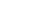is an intuitionistic Kripke frame
Kripke semantics
Kripke semantics is a formal semantics for non-classical logic systems created in the late 1950s and early 1960s by Saul Kripke. It was first made for modal logics, and later adapted to intuitionistic logic and other non-classical systems...

, and we define a model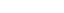in this frame by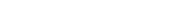This definition obeys the conditions on satisfaction of →: on one hand, if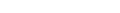, and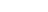is such that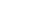and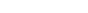, then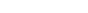by modus ponens. On the other hand, if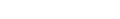, then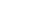by the deduction theorem
Deduction theorem
In mathematical logic, the deduction theorem is a metatheorem of first-order logic. It is a formalization of the common proof technique in which an implication A → B is proved by assuming A and then proving B from this assumption. The deduction theorem explains why proofs of conditional...

, thus the deductive closure of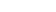is an elementsuch that,, and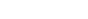.

Let A be any formula which is not provable in the calculus. Then A does not belong to the deductive closure X of the empty set, thus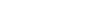, and A is not intuitionistically valid.

• SKI combinator calculus
SKI combinator calculus
SKI combinator calculus is a computational system that may be perceived as a reduced version of untyped lambda calculus. It can be thought of as a computer programming language, though it is not useful for writing software...

• B,C,K,W system
B,C,K,W system
The B, C, K, W system is a variant of combinatory logic that takes as primitive the combinators B, C, K, and W. This system was discovered by Haskell Curry in his doctoral thesis Grundlagen der kombinatorischen Logik, whose results are set out in Curry .The combinators are defined as follows:* B x...

• Fixed point combinator
Fixed point combinator
In computer science, a fixed-point combinator is a higher-order function that computes a fixed point of other functions. A fixed point of a function f is a value x such that x = f. For example, 0 and 1 are fixed points of the function f = x2, because 0 = 02 and 1 = 12...

• graph reduction machine
Graph reduction machine
A graph reduction machine is a special-purpose computer built to perform combinator calculations by graph reduction.Examples include the SKIM computer, built at the University of Cambridge Computer Laboratory, and the multiprocessor GRIP computer, built at University College London.- References...

• supercombinator
Supercombinator
A supercombinator is a mathematical expression which is fully bound and self-contained. It may either be a constant or a combinator where all the subexpressions are supercombinators....

s
• Lambda calculus
Lambda calculus
In mathematical logic and computer science, lambda calculus, also written as λ-calculus, is a formal system for function definition, function application and recursion. The portion of lambda calculus relevant to computation is now called the untyped lambda calculus...

and Cylindric algebra
Cylindric algebra
The notion of cylindric algebra, invented by Alfred Tarski, arises naturally in the algebraization of first-order logic with equality. This is comparable to the role Boolean algebras play for propositional logic. Indeed, cylindric algebras are Boolean algebras equipped with additional...

, other approaches to modelling quantification and eliminating variables
• To Mock a Mockingbird
To Mock a Mockingbird
To Mock a Mockingbird and Other Logic Puzzles: Including an Amazing Adventure in Combinatory Logic is a book by the mathematician and logician Raymond Smullyan. It contains many nontrivial recreational puzzles of the sort for which Smullyan is well-known...

• combinatory categorial grammar
Combinatory categorial grammar
Combinatory categorial grammar is an efficiently parseable, yet linguistically expressive grammar formalism. It has a transparent interface between surface syntax and underlying semantic representation, including predicate-argument structure, quantification and information structure.CCG relies on...

• Categorical abstract machine
Categorical abstract machine
The categorical abstract machine is a model of computation for programs that preserves the abilities of applicative, functional, or compositional style. It is based on the techniques of applicative computing.- Overview :...

• Applicative computing systems
Applicative computing systems
Applicative computing systems, or ACS are the systems of object calculi founded on combinatory logic and lambda calculus.The only essential notion which is under consideration in these systems is the representation of object. In combinatory logic the only metaoperator is application in a sense of...

• Hendrik Pieter Barendregt, 1984. The Lambda Calculus, Its Syntax and Semantics. Studies in Logic and the Foundations of Mathematics, Volume 103, North-Holland. ISBN 0-444-87508-5
• Field, Anthony J. and Peter G. Harrison, 1998. Functional Programming. . Addison-Wesley. ISBN 0-201-19249-7

| journal = Journal of Symbolic Logic
Journal of Symbolic Logic
The Journal of Symbolic Logic is a peer-reviewed mathematics journal published quarterly by Association for Symbolic Logic.Founded in 1936, the journal publishes articles on mathematical logic....

| volume = 55
| issue = 1
| pages = 90–105
| year = 1990
}}
• Hindley, J. R., and Seldin, J. P. (2008) λ-calculus and Combinators: An Introduction. Cambridge Univ. Press.
• Paulson, Lawrence C., 1995. Foundations of Functional Programming. University of Cambridge.
• Quine, W. V.
Willard Van Orman Quine
Willard Van Orman Quine was an American philosopher and logician in the analytic tradition...

, 1960 "Variables explained away", Proceedings of the American Philosophical Society 104:3:343–347 (June 15, 1960) at JSTOR. Reprinted as Chapter 23 of Quine's Selected Logic Papers (1966), pp. 227–235
• Moses Schönfinkel
Moses Schönfinkel
Moses Ilyich Schönfinkel, also known as Moisei Isai'evich Sheinfinkel , was a Russian logician and mathematician, known for the invention of combinatory logic.- Life :Schönfinkel attended the Novorossiysk University of Odessa, studying mathematics under Samuil Osipovich...

, 1924, "Über die Bausteine der mathematischen Logik," translated as "On the Building Blocks of Mathematical Logic" in From Frege to Gödel: a source book in mathematical logic, 1879–1931, Jean van Heijenoort
Jean Van Heijenoort
Jean Louis Maxime van Heijenoort was a pioneer historian of mathematical logic. He was also a personal secretary to Leon Trotsky from 1932 to 1939, and from then until 1947, an American Trotskyist activist.-Life:Van Heijenoort was born in Creil, France...

, ed. Harvard University Press, 1967. ISBN 0-674-32449-8. The article that founded combinatory logic.
• Sørensen, Morten Heine B. and Paweł Urzyczyn, 1999. Lectures on the Curry–Howard Isomorphism. University of Copenhagen and University of Warsaw, 1999.
• Smullyan, Raymond
Raymond Smullyan
Raymond Merrill Smullyan is an American mathematician, concert pianist, logician, Taoist philosopher, and magician.Born in Far Rockaway, New York, his first career was stage magic. He then earned a BSc from the University of Chicago in 1955 and his Ph.D. from Princeton University in 1959...

, 1985. To Mock a Mockingbird
To Mock a Mockingbird
To Mock a Mockingbird and Other Logic Puzzles: Including an Amazing Adventure in Combinatory Logic is a book by the mathematician and logician Raymond Smullyan. It contains many nontrivial recreational puzzles of the sort for which Smullyan is well-known...

. Knopf. ISBN 0-394-53491-3. A gentle introduction to combinatory logic, presented as a series of recreational puzzles using bird watching metaphors.
• --------, 1994. Diagonalization and Self-Reference. Oxford Univ. Press. Chpts. 17-20 are a more formal introduction to combinatory logic, with a special emphasis on fixed point results.
• Wolfengagen, V.E. Combinatory logic in programming. Computations with objects through examples and exercises. -- 2-nd ed. -- M.: "Center JurInfoR" Ltd., 2003. -- x+337 с. ISBN 5-89158-101-9.